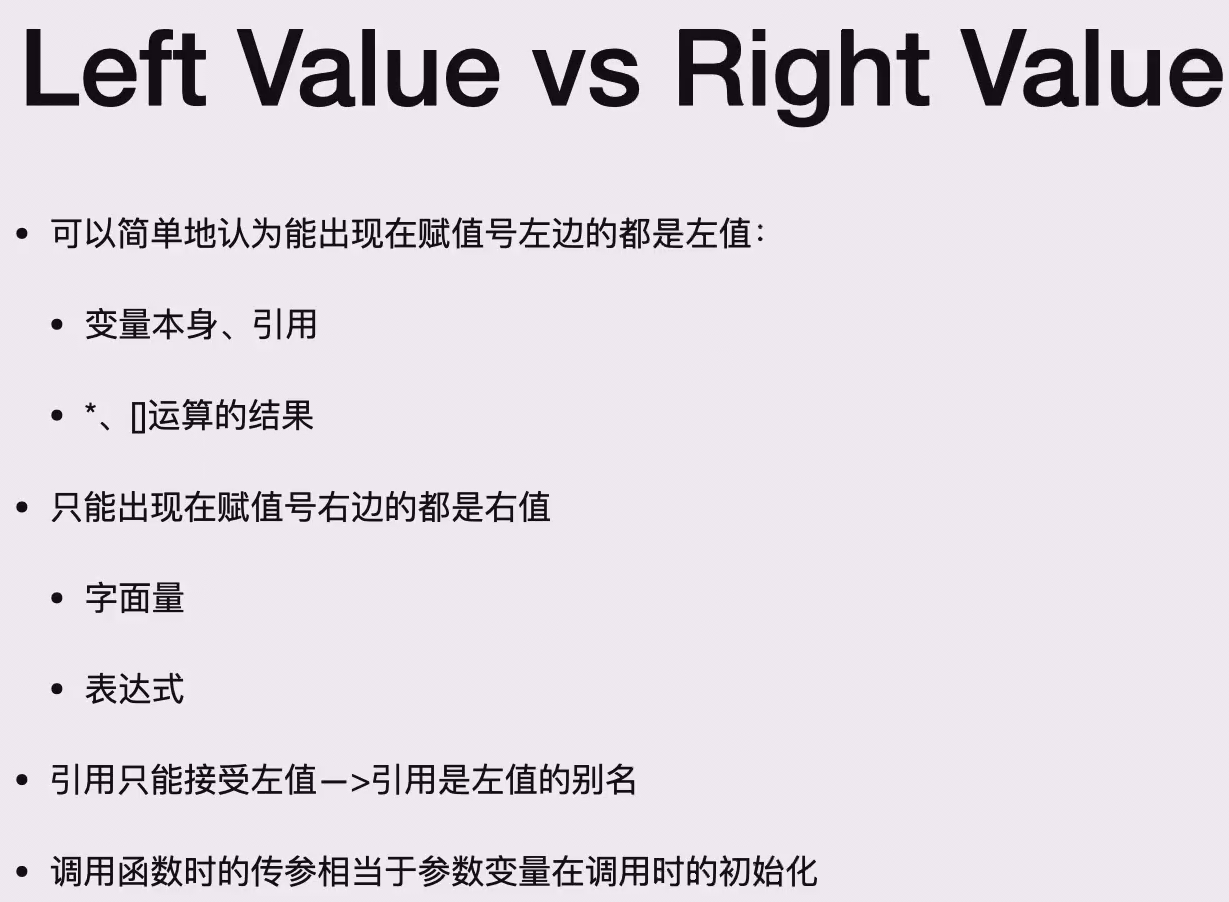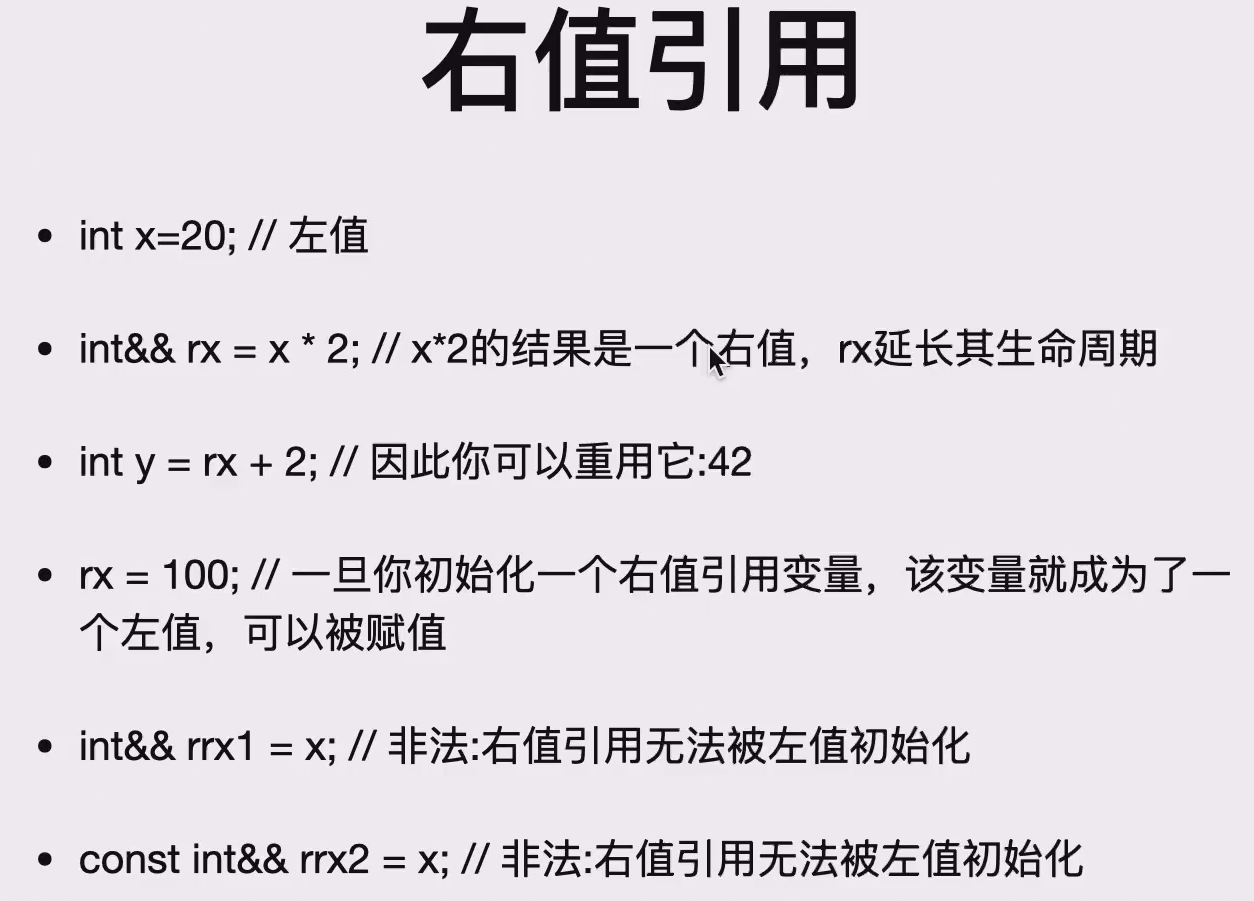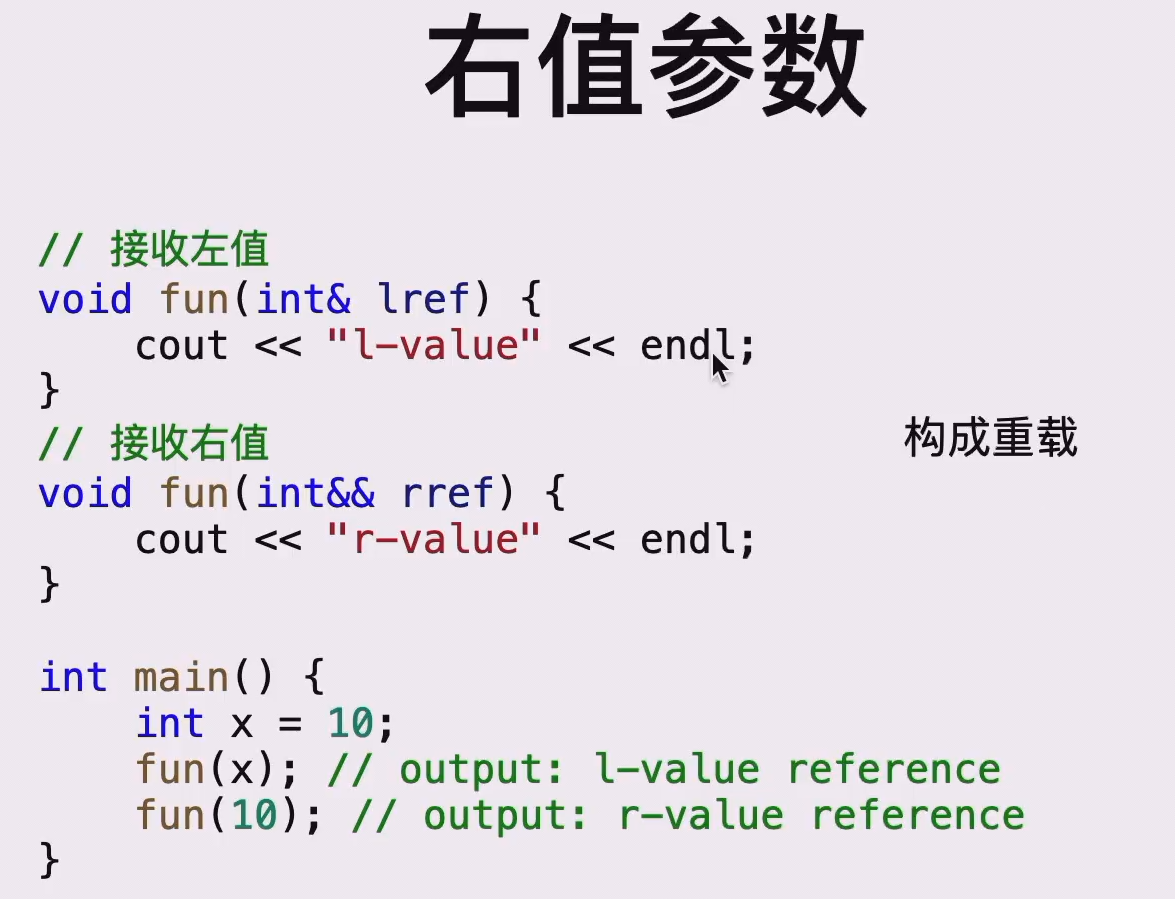# [pre] 前置与杂项¶

## [1.x] 引用reference¶

1.创建binding关系

typename & refname = name; 创建了 (typename)name 的引用 (reference)refname

• 一般来说(不考虑const+reference)能创建的引用的必须是个变量，而不能是个表达式、常量。
• 换句话说，只能给左值(left value)创建引用 特别的：
int x = 1, y = 10, *p = &x, &ref = *p;
// 此时 x = 1, y = 10, *p = 1, ref = 1

*p = 2;
// 此时 x = 2, y = 10, *p = 2, ref = 2

p = &y;
// 此时 x = 2, y = 10, *p = 10, ref = 2

• 引用在创建的时候必须初始化，即int &x;错误；int &x = y;正确。
• Bindings一旦建立，无法取消
• reference本质上就是受限的pointer
• reference不存在reference
• reference不存在pointer
• 定义int *p = &x;
• int&* ref = ...;非法，ref不能是一个引用的指针
• int*& ref = p;合法，ref是一个指针的引用
• 不存在reference数组

2.利用reference传参

void f(int &x){
x = 1;
}

int main(){
int p = 0;
// 此时 p = 0
f(p);
// 此时 p = 1
}


3.referenceconst

• int &refA = A;非法
• const int &refA = A;合法
• const int &refA = 1;合法
• 函数传参同理

## [2.x] 范围解析运算符resolver¶

• <Class Name>::<Function Name> 表示这个 <Function Name> 是不自由的，属于 <Class Name>的；
• ::<Function Name> 表示这个 <Function Name>是全局变量中的自由函数（变量同理）；
#include<iostream>
using namespace std;

class S{
public:
void f();
private:
int a;
};

int a;

void f(){
cout << "qwq\n";
}

void S::f(){ // 这里正在定义一个属于类S的的函数
++a;     // 这里对一个类S的成员变量a进行了自增操作
::f();   // 这里调用了一个全局下的自由函数
++::a;   // 这里对一个全局变量a进行了自增操作
cout << a << " " << ::a << "\n";
}

int main(){
S k;
k.f();
}

• 类的静态变量属于类不属于实例，不能通过正常的方式初始化，可以用过范围解析运算符在全局初始化
• 实际上，编译器将对象对静态变量的访问自动转换成了类对静态变量的访问。因此，建议始终使用className::staticVar访问静态变量_(xxjj在这里说的)_

int k(int x){ return x; }

char k(long x){ return 'a'; }

int main(){
cout << k(1L);   // 输出：a
cout << k(1);    // 输出：1
}


## [4.x] 函数的默认参数Default Argument¶

• 此时调用fun()可以省略存在默认参数的参数
• 但是不存在默认值的参数必须在存在默认值的参数前面
• void fun(int x, int a = 0);合法
• void fun(int x = 0, int a);非法
• 如果同时存在函数声明和函数body，则在这两部分中Default Argument只能在其中一个
// 合法
void fun(int x,int y);

void fun(int x = 1,int y = 2){
// ...
}

// 非法，因为x不能在y前
void fun(int x = 1,int y);

void fun(int x,int y = 2){
// ...
}

// 合法
void fun(int x = 1,int y = 2);

void fun(int x,int y){
// ...
}


## [5.x] 内联函数inline¶

• inline仅仅是对编译器的一个建议
• 🔗引用一下xxjj的文章
• 需要注意的是，inline是在每一个编译单元中进行的，所以这就导致了内联函数的原型应当能直接被调用该函数的文件访问到。
• eg.
• a.cpp中调用f();#include "b.h"
• b.h中声明inline void f();
• b.cpp中写明f()的body
• 结果就是会编译错误——需要将f()的body放到a.cpp或者b.h中才可以——因为在编译的时候a.cpp仅和b.h一起编译，并不知道f()的body在哪里，无法进行“内联”
• inline与 宏 很相似，但是有一些区别：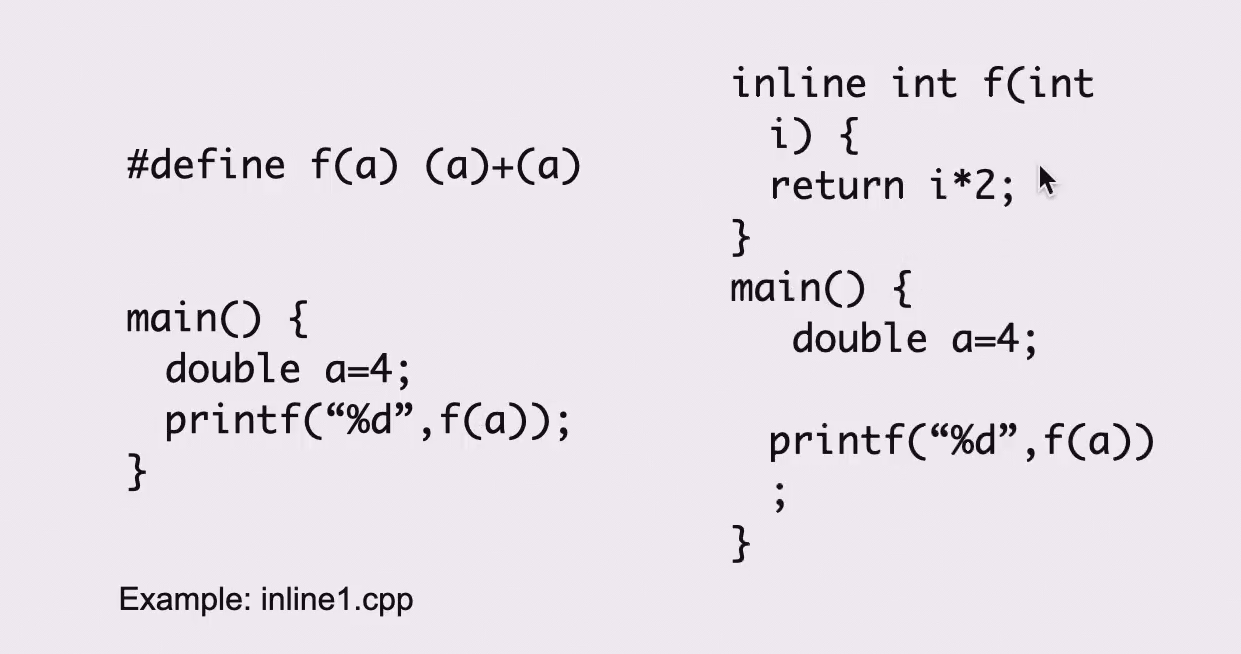• 做的事情是类似的，但用宏无法实现隐式类型转化
• 对编译器来说inline只是个建议，编译器未必会实现
• 在一个类里定义body的函数，它默认是inline函数
• 如果在类里没有定义body，那么该函数是内联函数当且仅当前面有inline关键词

## [6.x] const¶

const对数据的保护出现在编译时刻，如果强行通过某种手段获取其地址，仍然可以修改，编译器仅仅保证const修饰过的变量除了定义时，不能作为左值

• char * const p;修饰的是p
• const char *p;修饰的是*p
• char const *p;修饰的是*p
• const char * const p;*pp都被修饰
• const出现在*左侧，修饰的是*p
• const出现在*右侧，修饰的是p
• （若const char *p = &ch;，虽然通过*p无法更改指，但ch仍然可以修改，进一步体现这是个编译时刻行为）
•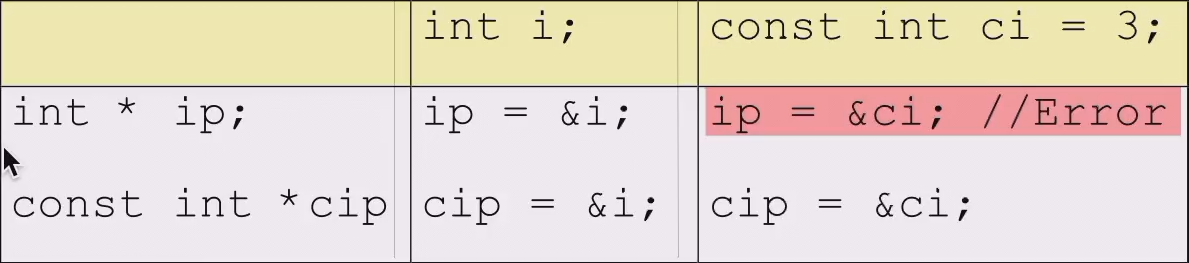• 在这些性质下，我们可以把一个非const的变量赋值给const变量（特别是函数传参），来控制指针的读写权限（可以用来减少代码写错的情况）
•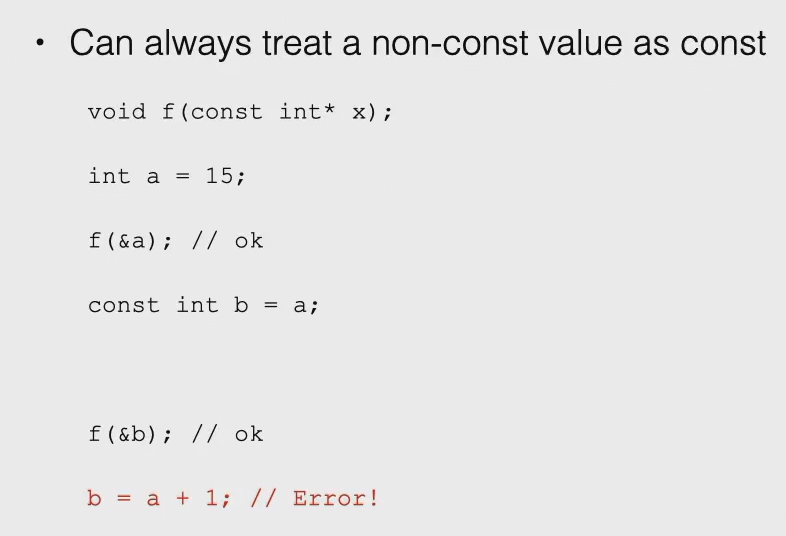• 一个被const修饰过的对象的成员函数只有在该函数也被const修饰过且经过编译器检测确实不修改成员变量的情况下才能执行
•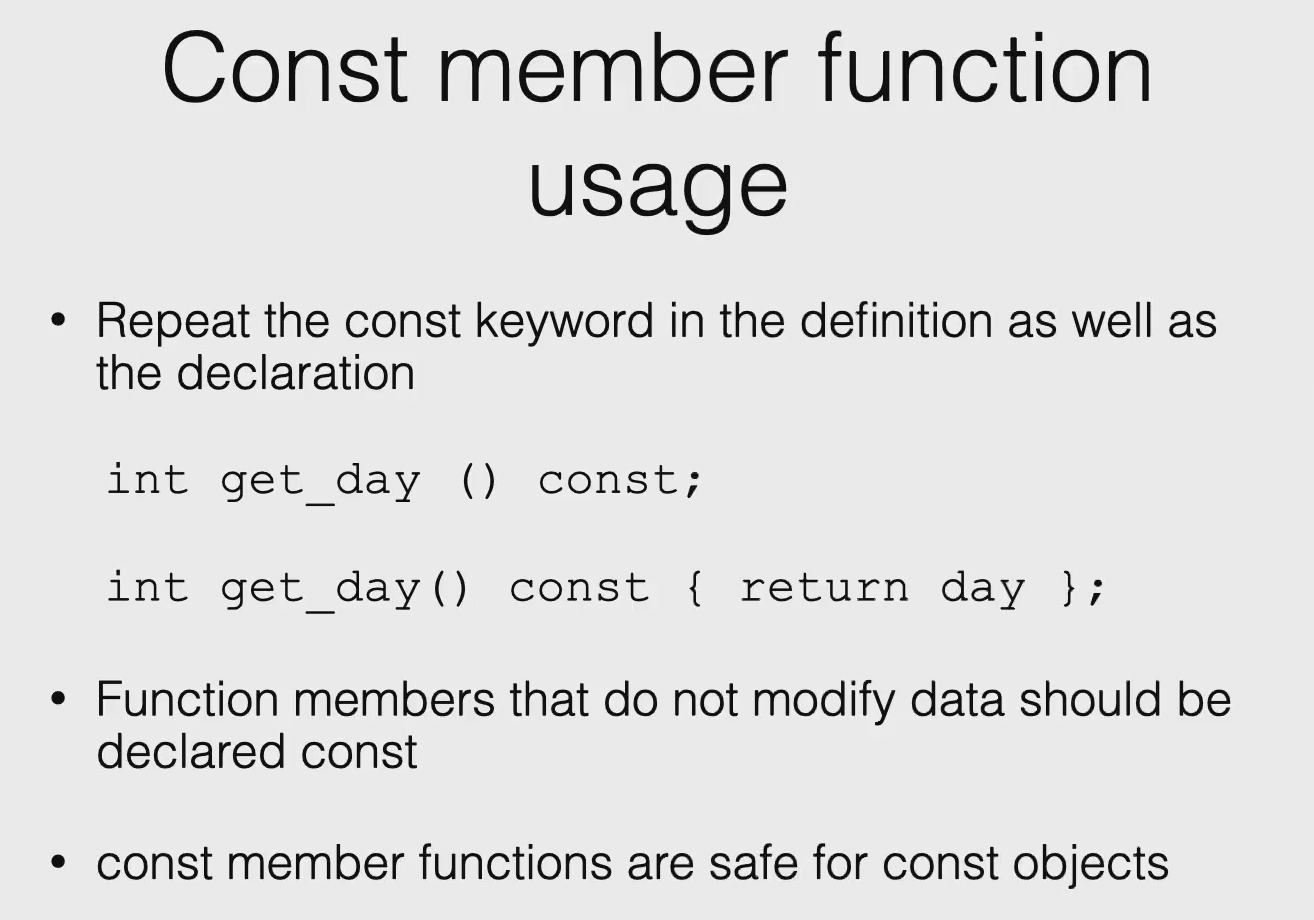• int A::fun() const;int A::fun();可以构成overload关系，因为这里的const实际上指的是隐藏的A *thisconst修饰，参数表不同，符合overload条件
• 当上述两个函数都存在时，当且仅当对象被const修饰才会调用前者
• 如果成员变量是const，那必须存在初始化方案

## [7.x] static¶

• 类的静态变量属于类不属于实例，不能通过正常的方式初始化，可以用过范围解析运算符在全局初始化
• 实际上，编译器将对象对静态变量的访问自动转换成了类对静态变量的访问。因此，建议始终使用className::staticVar访问静态变量_(xxjj在这里说的)_
• 类的静态函数不再具有this指针，也不能直接访问非静态成员变量或者非静态成员函数
• 一般情况下，如果要在class里声明static变量/函数，则只在.h文件中出现关键字，不要在.cpp中出现

## [8.x] 命名空间namespace & using关键字¶

namespace <Space Name>{
// 内容
}


• using namespace xxx;后可以省略<Space Name>::，但是要注意歧义问题
• using xxx::yyy;后仅仅对yyy来说可以省略<Space Name>::，同样要注意歧义问题
• 两个命名空间有相同函数/变量的时候，需要写明命名空间
• namespace x = y;表示可以用x来表示命名空间y，但是在此前y必须已经定义
• namespace里也可以有namespace，我们称之为 组合

## [9.x] 左值与右值&右值引用¶